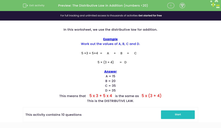# The Distributive Law in Addition (numbers <20)

In this worksheet, students use the distributive law in addition.Key stage:  KS 3

Curriculum topic:   Number

Curriculum subtopic:   Use Relationships Between Operations

Difficulty level:#### Worksheet Overview

In this worksheet, we use the distributive law for addition.

Example

Work out the values of A, B, C and D.

5 ×3 + 5×4 =
 A
+
 B
=
 C

5 × (3 + 4) =
 D

A = 15
B = 20
C = 35
D = 35

This means that    5 x 3 + 5 x 4   is the same as   5 x (3 + 4)

This is the DISTRIBUTIVE LAW.

### What is EdPlace?

We're your National Curriculum aligned online education content provider helping each child succeed in English, maths and science from year 1 to GCSE. With an EdPlace account you’ll be able to track and measure progress, helping each child achieve their best. We build confidence and attainment by personalising each child’s learning at a level that suits them.

Get started